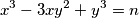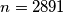### IMO Shortlist 1982 problem 16

Kvaliteta:
Avg: 0,0
Težina:
Avg: 0,0
Prove that if$n$ is a positive integer such that the equationhas a solution in integers$x,y$, then it has at least three such solutions. Show that the equation has no solutions in integers for$n=2891$.
Izvor: Međunarodna matematička olimpijada, shortlist 1982